# PHY 2049 Physics II n n Tutoring Center

• Slides: 14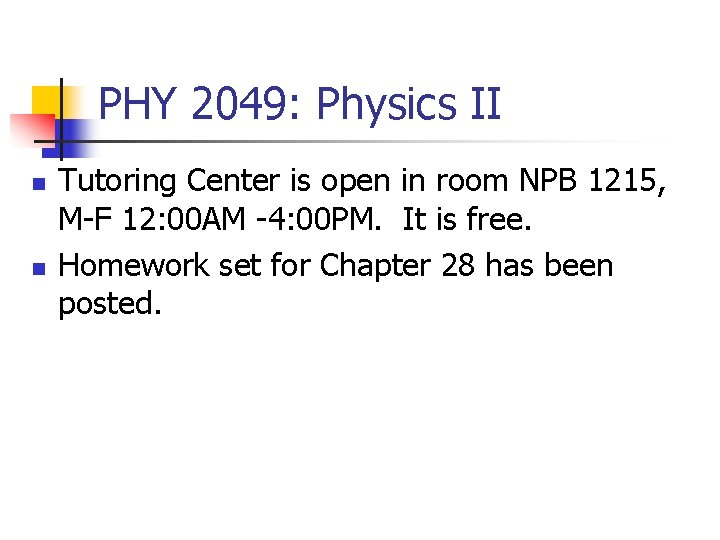PHY 2049: Physics II n n Tutoring Center is open in room NPB 1215, M-F 12: 00 AM -4: 00 PM. It is free. Homework set for Chapter 28 has been posted.Circuits, networks and all that n n Spread of information By Foot n n Spread of Christianity, St. Paul and his travels Buddhist missionaries By Electromagnetic waves. Connections (degrees of) n n n Telegraph, Radio, TV, cell phones By Internet Connections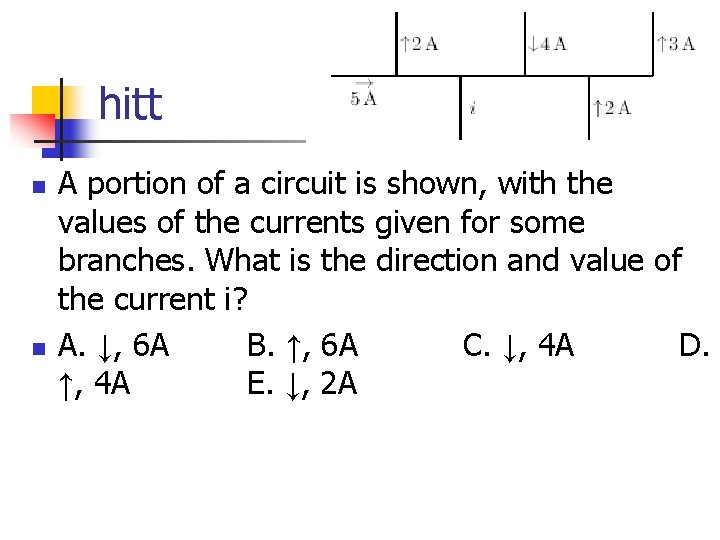hitt n n A portion of a circuit is shown, with the values of the currents given for some branches. What is the direction and value of the current i? A. ↓, 6 A B. ↑, 6 A C. ↓, 4 A D. ↑, 4 A E. ↓, 2 AElectrical circuits n n Branches and junctions Kirchhoff's Rules n n All current coming to a junction equals the total current leaving the junction. (charge conservation) Around any closed loop, the total voltage drop vanishes. (work around a closed loop)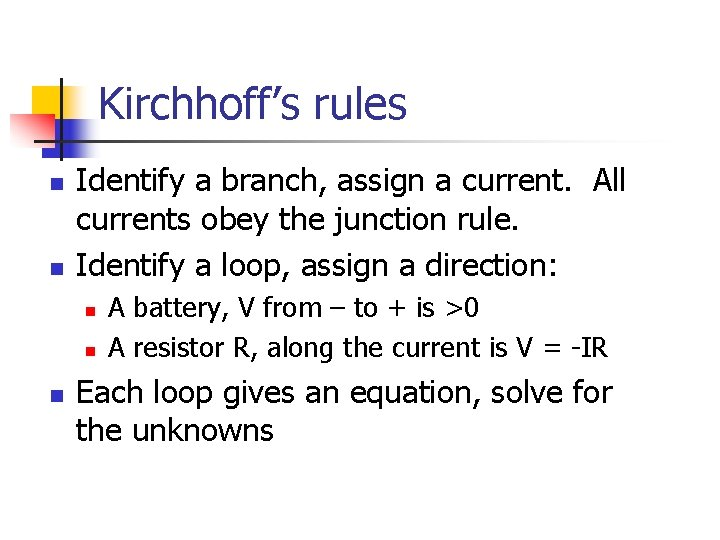Kirchhoff’s rules n n Identify a branch, assign a current. All currents obey the junction rule. Identify a loop, assign a direction: n n n A battery, V from – to + is >0 A resistor R, along the current is V = -IR Each loop gives an equation, solve for the unknowns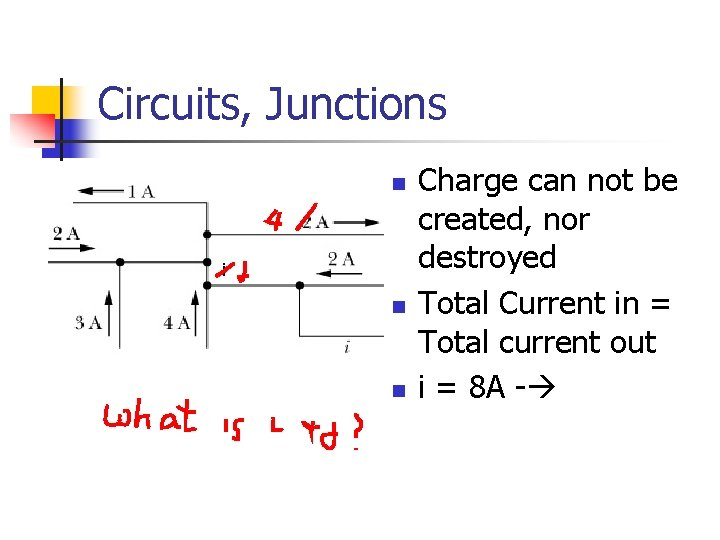Circuits, Junctions n i n n Charge can not be created, nor destroyed Total Current in = Total current out i = 8 A -Loop calculations n n R 2 and R 3 are parallel. The net is in series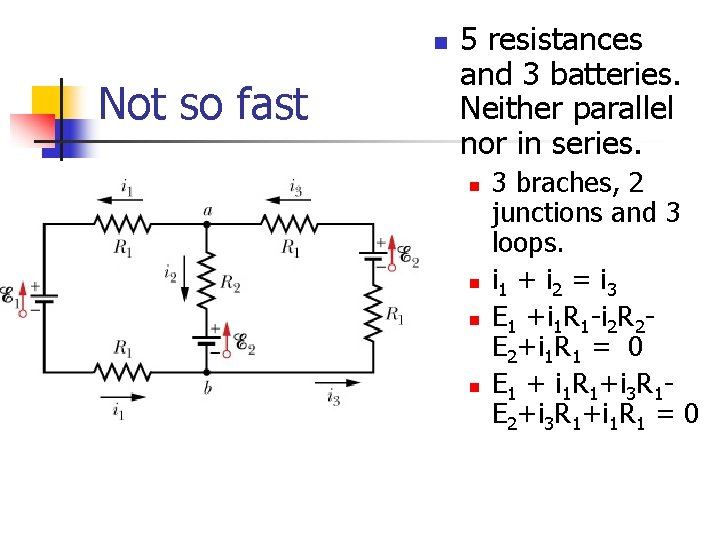n Not so fast 5 resistances and 3 batteries. Neither parallel nor in series. n n 3 braches, 2 junctions and 3 loops. i 1 + i 2 = i 3 E 1 +i 1 R 1 -i 2 R 2 E 2+i 1 R 1 = 0 E 1 + i 1 R 1+i 3 R 1 E 2+i 3 R 1+i 1 R 1 = 0n What is i? All V = 10 Volts and all R = 4 Ohmsj k n n What is i? All V = 10 Volts and all R = 4 Ohms? What is j? What is k?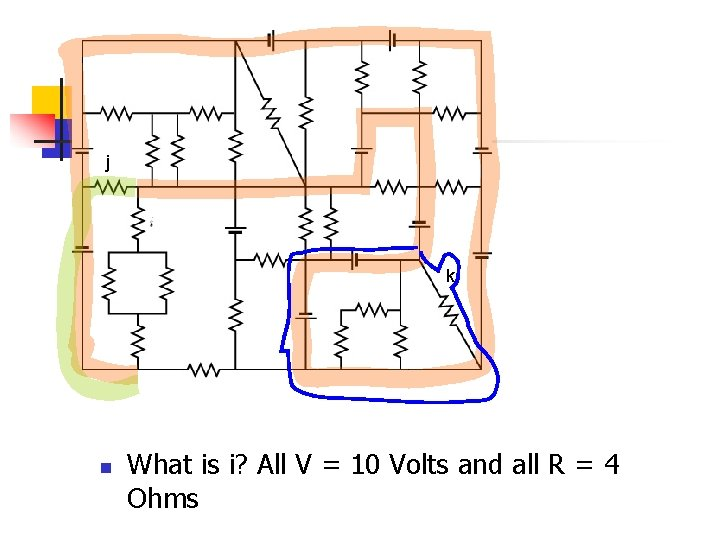j k n What is i? All V = 10 Volts and all R = 4 OhmsR = 4 Ω, V = 4 V, what is the current through R?R = 4 Ω, V = 4 V, what is the current through R? 8 = 4 I => I = 2 A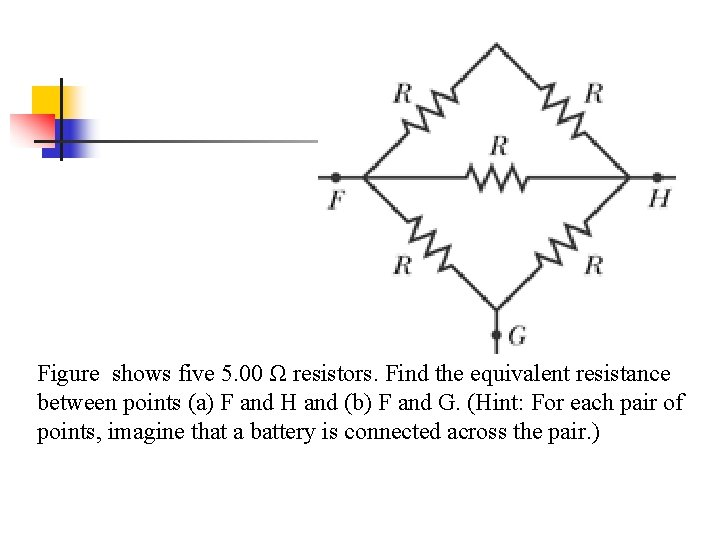Figure shows five 5. 00 Ω resistors. Find the equivalent resistance between points (a) F and H and (b) F and G. (Hint: For each pair of points, imagine that a battery is connected across the pair. )